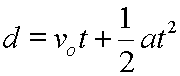# Introduction to the Displacement and Acceleration Equation

This equation relates displacement, original velocity, constant acceleration, and time:Displacement equals the original velocity multiplied by time plus one half the acceleration multiplied by the square of time.

Here is a sample problem and its solution showing the use of this equation:

An object is moving with a velocity of 5.0 m/s. It accelerates constantly at 2.0 m/s/s, (2 m/s2), for a time period of 3.0 s. What is its displacement during this acceleration?

 d = vot + (1/2)at2 Start here. d = (5.0 m/s)(3.0 s) + (1/2)(2.0 m/s/s)(3.0 s)2 Plug in values with units. d = 15 + 9 Do some intermediate mathematics. d = 24 m Come up with a final answer.

So, this object moved 24 meters during the 3.0 seconds of the acceleration.

More:

One way to understand the origin of this equation is to examine a constant acceleration demonstrated on a velocity vs. time graph.

Using algebra this equation can be rearranged and solved for any of the variables present in it.

There are equivalent alternate symbols for the quantities in this equation.

Custom Search